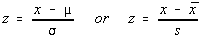Standard Scores (z-scores)

The standard score is obtained by subtracting the mean and dividing the difference by the standard deviation. The symbol is z, which is why it's also called a z-score.The mean of the standard scores is zero and the standard deviation is 1. This is the nice feature of the standard score -- no matter what the original scale was, when the data is converted to its standard score, the mean is zero and the standard deviation is 1.

Percentiles, Deciles, Quartiles

Percentiles (100 regions)

The kth percentile is the number which has k% of the values below it. The data must be ranked.

1. Rank the data
2. Find k% (k /100) of the sample size, n.
3. If this is an integer, add 0.5. If it isn't an integer round up.
4. Find the number in this position. If your depth ends in 0.5, then take the midpoint between the two numbers.

It is sometimes easier to count from the high end rather than counting from the low end. For example, the 80th percentile is the number which has 80% below it and 20% above it. Rather than counting 80% from the bottom, count 20% from the top.

Note: The 50th percentile is the median.

If you wish to find the percentile for a number (rather than locating the kth percentile), then

1. Take the number of values below the number
3. Divide by the total number of values
4. Convert it to a percent

Deciles (10 regions)

The percentiles divide the data into 100 equal regions. The deciles divide the data into 10 equal regions. The instructions are the same for finding a percentile, except instead of dividing by 100 in step 2, divide by 10.

Quartiles (4 regions)

The quartiles divide the data into 4 equal regions. Instead of dividing by 100 in step 2, divide by 4.

Note: The 2nd quartile is the same as the median. The 1st quartile is the 25th percentile, the 3rd quartile is the 75th percentile.

The quartiles are commonly used (much more so than the percentiles or deciles). The TI-82 calculator will find the quartiles for you. Some textbooks include the quartiles in the five number summary.

Hinges

The lower hinge is the median of the lower half of the data up to and including the median. The upper hinge is the median of the upper half of the data up to and including the median.

The hinges are the same as the quartiles unless the remainder when dividing the sample size by four is three (like 39 / 4 = 9 R 3).

The statement about the lower half or upper half including the median tends to be confusing to some students. If the median is split between two values (which happens whenever the sample size is even), the median isn't included in either since the median isn't actually part of the data.

Example 1: sample size of 20

The median will be in position 10.5. The lower half is positions 1 - 10 and the upper half is positions 11 - 20. The lower hinge is the median of the lower half and would be in position 5.5. The upper hinge is the median of the upper half and would be in position 5.5 starting with original position 11 as position 1 -- this is the original position 15.5.

Example 2: sample size of 21

The median is in position 11. The lower half is positions 1 - 11 and the upper half is positions 11 - 21. The lower hinge is the median of the lower half and would be in position 6. The upper hinge is the median of the upper half and would be in position 6 when starting at position 11 -- this is original position 16.

Five Number Summary

The five number summary consists of the minimum value, 1st quartile, median, 3rd quartile, and maximum value. Some textbooks use the hinges instead of the quartiles.

Box and Whiskers Plot

A graphical representation of the five number summary. A box is drawn between the lower and upper hinges with a line at the median. Whiskers (a single line, not a box) extend from the hinges to lines at the minimum and maximum values.

Interquartile Range (IQR)

The interquartile range is the difference between the third and first quartiles. That's it: Q3 - Q1

Outliers

Outliers are extreme values. There are mild outliers and extreme outliers. The Bluman text does not distinguish between mild outliers and extreme outliers and just treats either as an outlier.

Extreme Outliers

Extreme outliers are any data values which lie more than 3.0 times the interquartile range below the first quartile or above the third quartile. x is an extreme outlier if ...

x < Q1 - 3 * IQR

or

x > Q3 + 3 * IQR

Mild Outliers

Mild outliers are any data values which lie between 1.5 times and 3.0 times the interquartile range below the first quartile or above the third quartile. x is a mild outlier if ...

Q1 - 3 * IQR <= x < Q1 - 1.5 * IQR

or

Q1 + 1.5 * IQR < x <= Q3 + 3 * IQR